Computational & Technology Resources
an online resource for computational,
engineering & technology publications
Civil-Comp Proceedings
ISSN 1759-3433
CCP: 74
PROCEEDINGS OF THE SIXTH INTERNATIONAL CONFERENCE ON THE APPLICATION OF ARTIFICIAL INTELLIGENCE TO CIVIL AND STRUCTURAL ENGINEERING
Edited by: B.H.V. Topping and B. Kumar
Paper 25

Pollution Modelling for Hong Kong Downtown Area Using Principal Component Analysis and Artificial Neural Networks

W.Z. Lu+, H.Y. Fan+*, A.Y.T. Leung+, W.J. Wang+*, S.M. Lo+ and J.C.K. Wong*

+Department of Building & Construction, City University of Hong Kong, PR China
*Xi'an Jiaotong University, Xi'an, PR China

Full Bibliographic Reference for this paper
W.Z. Lu, H.Y. Fan, A.Y.T. Leung, W.J. Wang, S.M. Lo, J.C.K. Wong, "Pollution Modelling for Hong Kong Downtown Area Using Principal Component Analysis and Artificial Neural Networks", in B.H.V. Topping, B. Kumar, (Editors), "Proceedings of the Sixth International Conference on the Application of Artificial Intelligence to Civil and Structural Engineering", Civil-Comp Press, Stirlingshire, UK, Paper 25, 2001. doi:10.4203/ccp.74.25
Keywords: environmental, modelling, neural network, pollutant, principal component analysis, respirable suspended particulate.

Summary
Modeling of the pollutants' concentrations comprises an important part in the field of atmospheric environment research. Neural network (NN) modeling is regarded as a reliable and cost-effective method to achieve such prediction task. This paper is a pioneer study of using combined principal component analysis (PCA) technique and neural network method to forecast the pollutants' concentration in down area of Hong Kong. The study is based on the data obtained at the Causeway Bay Roadside Gaseous Monitory Station, one of the eleven gaseous monitory stations established by HKEPD across Hong Kong area. The station recorded for the whole year of 1999 the hourly local concentrations of only six pollutants, i.e., nitric oxide (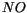), nitrogen dioxide (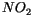), nitrogen oxides (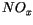), carbon monoxide (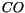), sulphur dioxide (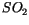) and respirable suspended particles (RSP). So far, the study reported here only emphasizes on modeling the pollutant concentrations in the local area on these available data.

The data of the six pollutants are first statistically analyzed to obtain the average variation characters of the pollutants' concentrations in the periods of a day, a week and a month during the period of 1999. Some regular features in variations of these six pollutants have been found.

The principal component analysis is then used to reduce and orthogonalize input variables of neural network model, which is established for forecasting the pollutants' concentrations. Principal component analysis is regarded as one of the main statistical tools for linearly reducing the dimensionality of a set of measurements while retaining as much information as possible about the original measurements. To use the principal component analysis, we calculate the covariance matrix of the studied data for all the six variables, i.e., the concentrations of six pollutants. The eigenvalues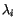, i =1,...,6, and eigenvectors of the covariance matrix are then computed. The resultant eigenvalues are listed as: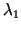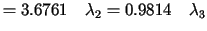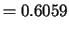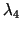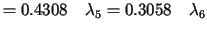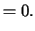In the above eigenvalues, the sixth one , this fact indicates that the sixth component almost has no contribution to the information of the studied data. Consequently, five new variables are then defined instead of the original six variables through projecting the variables onto the subspace spanned by the first five principal components. The new five variables so obtained are taken as input variables to predict the RSP concentrations. Such treatment of variables has two advantages. The first advantage is that the numbers of input variables used in a prediction process can be reduced, so that the structure of predictor and the computational cost can be reduced as well. The second one is that orthogonal input variables for neural networks can be obtained. Such orthogonalization can make a neural network easily be trained in general.

The multi-layer feed-forward neural networks are selected as the predictors in this application. A multi-layer feed-forward neural network consists of a series of simple interconnected neurons, or, nodes, which is a model representing a nonlinear mapping between input and output vectors. The multi-layer feed-forward neural networks have the ability to learn through training. During the training, a multi-layer feed-forward neural network is repeatedly presented with the training data and the weights in the network are adjusted from time to time till the desired input-output mapping occurs. This procedure results in the "encoding" of the properties of system to be mapped in the different parts of the neural network. If, after the training is completed, the multi-layer feed-forward neural networks are presented with an input vector, which is not belonging to the training pairs, it will simulate the system and produce a proper output vector.

In this study, two forecasting cases are implemented: the first case is predicting the daily mean RSP concentrations in advance of one day; while the second one is predicting the hourly RSP concentrations in advance of 24 hours. For both cases, the multi-layer feed-forward neural networks used has been structured with the form of "5-5-5-1" (i.e., the input layer with five neurons, both hidden layers with four neurons respectively, and the output layer with one neuron). In this network, the input neurons are used to present the five input variables, i.e., reduced from the original six pollutant variables through principal component analysis. The output of the networks represents the value of RSP concentrations to be predicted. The BP algorithm, the most commonly used one of the learning algorithms for multi-layer feed-forward neural network, is applied to train the neural networks.

The numerical simulations results for the two forecasting cases show that the neural network can well predict the RSP concentrations in subsequent time periods that are not belong to the training data set, except in a small neighborhoods of some maximum or minimum points among the testing data. Generally, it can be seen that the established neural networks have a good ability to forecast the concentrations of RSP. Alternatively, the proposed method can be used in forecasting the other pollutant's levels almost without further adaptation.

purchase the full-text of this paper (price £20)